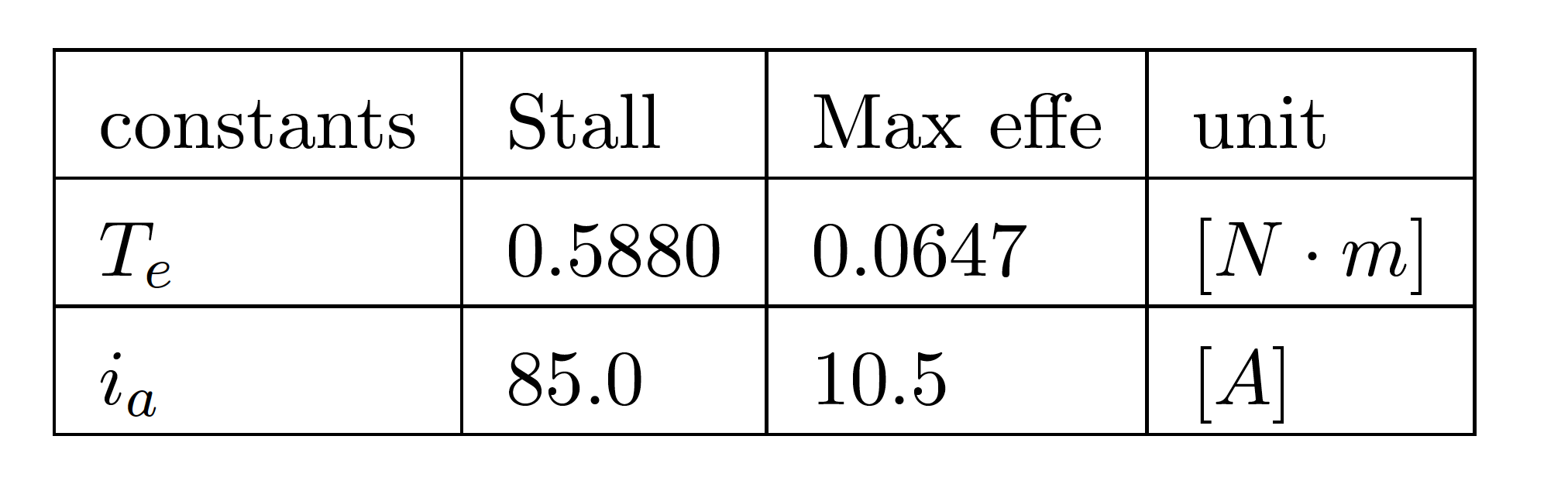# Background

Most of the driving force on robotics comes from an electric DC-motor (in this case permanent magnet). In order to use the DC-motor in the simulations, mathematical modelling has to be done, in order to yield the relations between the current, voltage and rotational speed. Here I will show how to setup model of a DC motor, parameter estimation of several constants of the DC-motor is also done.

# Mathematiclal ModellingFig. DC Motor Model

## Electrical Characteristics

By using Kirchoff’s voltage law in the DC-motor model according to fig. DC motor Model following equation is derived,$V_{in} - V_{Ra} -V_{La} -V_{emf} = 0$

Where,$V_{in}$,$V_{R_a}$,$V_{L_a}$ and$V_{emf}$ is the input-voltage, voltage over the armature resistance, voltage drop over the armature inductance and voltage induced by the coil respectively. The equations for the voltage parts in the DC-motor are,$V_{R_a} = i_a \cdot R_a$$V_{La} = L_a \cdot \frac{d}{dt}i_a$$V_{emf} = K_e \cdot \dot{\theta}_{a}$

Substituting first equation with${V_{Ra}}$,${V_{La}}$ and${V_{emf}}$ yields following differential equation,$V_{in} -i_a \cdot R_a -L_a \cdot \frac{d}{dt}i_a -K_e \cdot \dot{\theta}_{a} = 0$$\frac{d}{dt}i_a = \frac{1}{L_a} \cdot ( i_a \cdot R_a + K_e \cdot \dot{\theta}_{a} - V_{in} )$

(Differential Voltage equation)

## Mechanical Characteristics

By torque balance (energy balance) in the system the mechanical equations can be stated.$T_e -T_{\ddot{\theta}_{a}} -T_{b} -T_L=0$ (3.7)

Where$T_e$ is the electromagnetic torque.$T_{\dot{\theta}_a}$, is torque generated from the rotational acceleration of the rotor.$T_b$, is the torque due to the friction and angular velocity in the motor.$T_L$ is the torque of the mechanical load (external load). Equations for the first three parts are,$T_e = K_t \cdot i_a$ (3.8)$T_{\ddot{\theta}_{a}} = J_m \cdot \frac{d}{dt}{\dot{\theta}_{a}}$ (3.9)$T_{b} = b_m \cdot \dot{\theta}_{a}$ (3.10)

Substituting equation (3.7) with (3.8), (3.9) and (3.10) yields following differential

equation,$V_{in} -i_a \cdot R_a -L_a \cdot \frac{d}{dt}i_a -K_e \cdot \dot{\theta}_{a} = 0$$\frac{d}{dt}i_a = \frac{1}{L_a} \cdot ( i_a \cdot R_a + K_e \cdot \dot{\theta}_{a} - V_{in} )$

## Identifying parameters

By using the data sheet of the motor Maibuschi RS550VC, note that unknown parameter can be estimated. Note that the external load is zero during the estimation of the parameters$T_L=0$

### Torque-constant (Kt)

Usage of the stated equation the torque-constant$K_t$ and the back-wmf-constant$K_e$.

From equation ($T_e$ ), the torque-constant can be derived. Using both the data of the stall- , max-efficiency torque and current. The mean-value of this two gives a valid approximation of the torque-constant.$K_t = T_e/i_a$

Rewriting the equation first with$T_e=T_{stall}$ and$i_a=i_{stall}$. Where$T_{stall}$ is the stall torque form the data-sheet and $i_{stall}$ is the stall-current from the data-sheet, and then with$T_e=T_{effe}$ and$i_a=i_{effe}$, which is the max-efficiency, -torque and -current respectively. This yields,$K_{t_{stall}} = T_{stall}/i_{stall}$

and then with max-efficiency, -current and -torque.$K_{t_{effe}} = T_{effe}/i_{effe}$$K_t = \frac{K_{t_{stall}} + K_{t_{effe}}}{2}$

Table of the parameters that is used, (taken from the data-sheet) of Maibuschi RS550VCWith the stated equations and given parameters the \textit{torque-constant} is calculated to be,$K_t=0.0065$

### Back EMF-constant (Ke)

Using equation${V_emf}$ the Back-emf-constant can be calculated,$K_e = \frac{V_{emf}}{\dot{\theta}_{a}}$

In order to use$ke_{id}$ the$V_{emf}$ must be identified. In the data sheet, the non-load -speed and -current and the nominal voltage is used.$V_{emf} = V_{nominal} - (R_m \cdot i_{non-Load})$

The internal resistance is measured to be,$R_m =0.873$.The calculated back-wmf constant is,$K_e=0.0064$

Calculated and measured parameters. Note that the friction is yield by iteration in the simulation model (gray-box analysis), due to the fact that the maximum angular velocity at non-load is in the data-sheet.Now that the mathematical model is made , and the differential equations are stated it is time to start building the Simulink Model so that verification can be done.

From the differential equations it is mor or less straight forward to build the model. The image below shows the model that I have built.Running a simulation with non load of the DC motor.